### 6.7.37.1 Outline of the node

This node performs Voice Activity Detection (VAD) on multichannel speech waveform data using the ZeroCross method.

It can be used, for example, to detect speech segments independently and simultaneously on each microphone channel of an audio device with multiple handheld microphones.

It can also be used to extract a single microphone channel from a microphone array and compare its speech recognition performance with that of the isolated sound.

### 6.7.37.2 Necessary files

No files are required.

### 6.7.37.3 Usage

When to use

This node can know the Voice Activity of each channel of the multichannel audio waveform data to some extent. ZeroCross method is used as the detection for the voice activity. The voice activity detection result output from this node (provided as the same Source type as the localization result) is used for feature extraction in the latter stage.

Typical connection

Figure 6.149 shows an example of connection of VADZC ,

The input is multi-channel acoustic signal data of type Matrix<float> , output from AudioStreamFromWave , AudioStreamFromMic , etc. The output is the detected acoustic signal data of type Map<int, ObjectRef> (ObjectRef is of type Vector<float> ) and the result of the audio segment detection of type Vector<ObjectRef> (ObjectRef is of type Source ). Acoustic signal data is an input to MultiFFT or SaveWavePCM, sound source information is an input to Client or SaveWavePCM etc.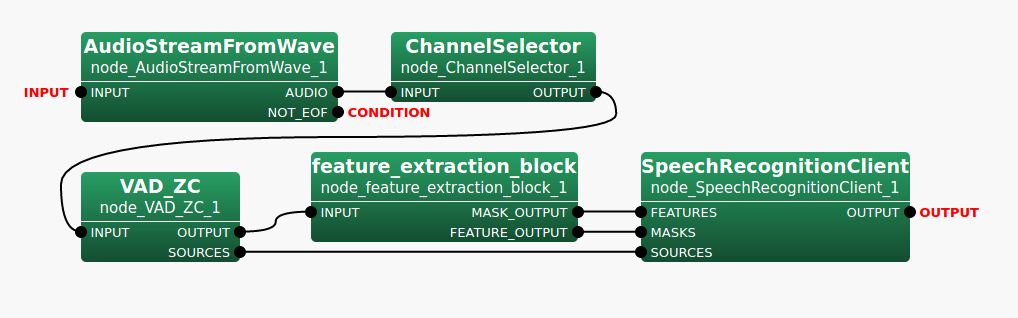Figure 6.149: Example of a connection of VADZC

### 6.7.37.4 Input-output and property of the node

Input

INPUT

: Matrix<float>  or Map<int, ObjectRef>  types. Multichannel speech waveform data. If the matrix size is $M \times L$, $M$ indicates the number of channels and $L$ indicates the sample numbers of waveforms. $L$ must be equal to the parameter LENGTH.

Output

OUTPUT

: Map<int, ObjectRef>  type. The PCM data detected in the audio segment. The Object part will be of type Vector<float> .

SOURCES

: Vector<ObjectRef>  type. The ID (channel number and audio index) that is uniquely assigned to the audio segment detection result. ObjectRef is Source and $number of microphones \times voice index + microphone index$ is stored in the ID. The rest of the values are dummies.

Parameter

Table 6.144: Parameter list of VADZC
 Parameter name Type Default value Unit Description ADVANCE 160 [pt] Shift length of frame. LENGTH 512 [pt] Number of samples of the frame. CHANNEL_COUNT 0 [ch] Number of input channels. SAMPLING_RATE 16000 [Hz] Sampling frequency. STRIP_ZERO true [pt] Enable / disable of Amplitude 0 frame removal function. ZERO_MEAN false Enable / disable Direct Current (DC) component removal function. LEVEL_THRESHOLD 2000 Threshold of soundless section cut(amplitude level) ZEROCROSS_THRESHOLD 60 Threshold of soundless section cut(Zero crossing number) HEAD_MARGIN 300 [ms] Margin at the beginning of the audio section. TAIL_MARGIN 400 [ms] Margin at the end of the audio section.

: int  type. Default value is 160. Specify the shift length of the frame.

LENGTH

: int  type. Default value is 512. Specify the number of samples of frame. It must be equal to the number of columns of Matrix<float> input to the INPUT pin. If it does not match, an exception is raised.

CHANNEL_COUNT

: int  type. Default value is 0. Specify the number of channels. It must be equal to the number of rows of Matrix<float> input to the INPUT pin. If it does not match, an exception is raised.

SAMPLING_RATE

: int  type. Default value is 16000. Specify the sampling rate.

STRIP_ZERO

: bool  type. Default value is true. Specify Enables(true)/disables(false) the function to exclude frames with a continuous amplitude of 0 in the audio waveform. Currently not used.

ZERO_MEAN

: bool  type. Default value is false. Enables(true) or disables(false) the function to remove the direct current (DC) component from the audio waveform. If the function is enabled by true, the DC component is calculated from the samples in the first frame and the DC offset of each frame is removed. It is processed before the speech segment detection (on the input buffer).

LEVEL_THRESHOLD

: float  type. Default value is 2000. Specify the silence cut threshold according to the amplitude level. The default value of 2000 is set as a guideline when a 16 bit signed integer of 0 - 32767 is input. To take into account the scaling of floating point numbers by MultiGain nodes etc., it is possible to set them as float .

ZEROCROSS_THRESHOLD

: int  type. Default value is 60. Specify the silence cut threshold in zero-crossings per second.

: int  type. Default value is 300. Specify the margin at the beginning of the audio section in milliseconds.

TAIL_MARGIN

: int  type. Default value is 400. Specify the margin at the end of the audio section in milliseconds.

### 6.7.37.5 Details of the node

About the condition to detect the voice section:

A frame in which both the amplitude level and the number of zero crossings of the acoustic signal exceed a threshold specified by a parameter is defined as a voice section start. In addition, as shown in Figure 6.150, margins can be provided at the beginning and end of the section.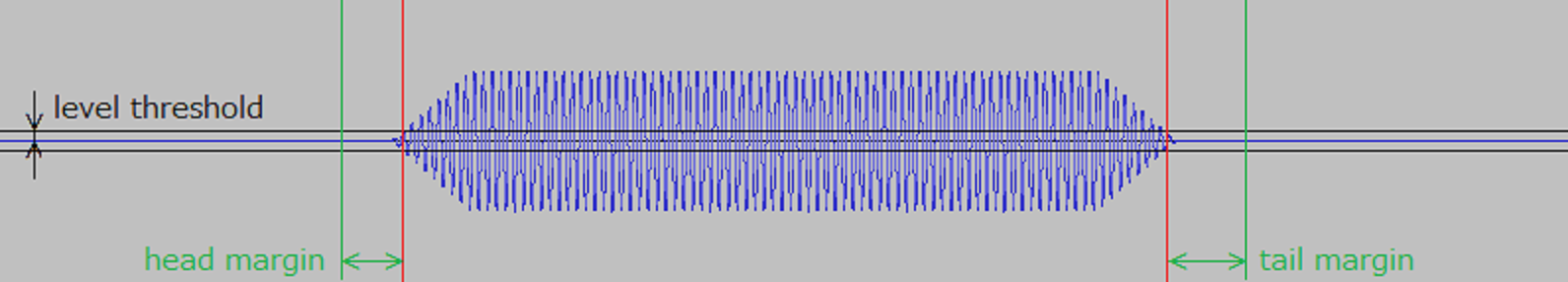Figure 6.150: Voice section detection

As an example, when LEVEL_THRESHOLD is 2000 and ZEROCROSS_THRESHOLD is 60, the audio interval is not detected under the following two conditions.

Figure 6.151 shows an example in which a 440 [Hz] sine wave with an amplitude level of about $\pm {1600}$ fades in and out. It indicates that the number of zero crossings is sufficient, but it is not detected because the amplitude level is less than LEVEL_THRESHOLD.

Figure 6.152 is an example of a 22 [Hz] sine wave with an amplitude level of about $\pm {26000}$ fading in and out. It shows that the amplitude level is sufficient, but it is not detected because the number of zero crossings is less than ZEROCROSS_THRESHOLD. (When the Sin wave is less than 30 [Hz], the number of zero crossings is less than 60).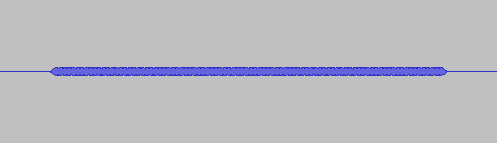Figure 6.151: Example of undetected due to insufficient amplitude level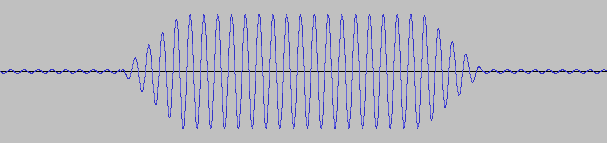Figure 6.152: Example of undetected due to insufficient number of zero crossings

About the Remove Function for Amplitude 0 Frames:

The STRIP_ZERO parameter for the Amplitude 0 Frame Removal function exists, but is not used and the setting value is ignored. The STRIP_ZERO parameter may be removed in a future version.

About Direct Current (DC) component removal function:

Figure 6.153 shows an example of removing a direct current (DC) component when the input acoustic signal contains a direct current (DC) component.

The upper part of the figure shows the input acoustic signal. The acoustic signal is a fade-in and fade-out of a 440 [Hz] Sin wave with an amplitude level of about $\pm {16000}$, but it is an example in which a direct current (DC) component is constantly included around + 6500.

The middle part of the figure shows the direct current (DC) component to be removed, and the direct current (DC) component of about + 6500 calculated using the first frame after the input of the acoustic signal is started is continuously removed in the subsequent frames.

The lower part of the figure shows the acoustic signal of the object for which voice section detection is actually performed, and it is shown that the zero crossing number can be correctly obtained by removing the DC component.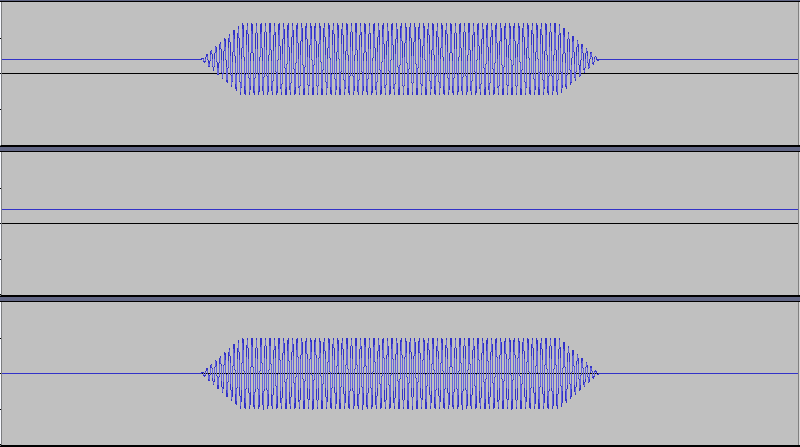Figure 6.153: Removal of the DC component (top: input acoustic signal, middle: DC component to be removed, bottom: acoustic signal to be VAD)